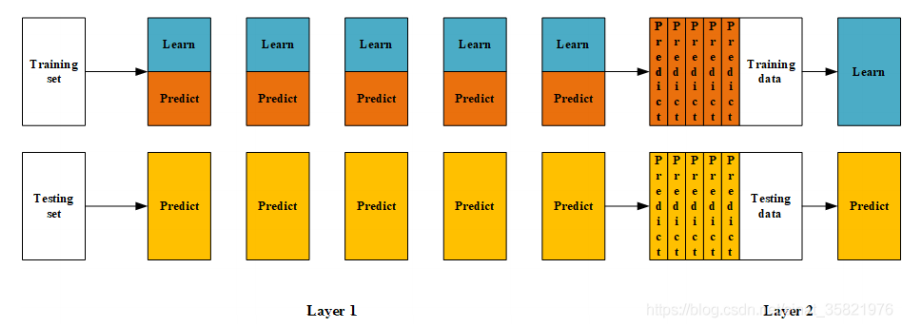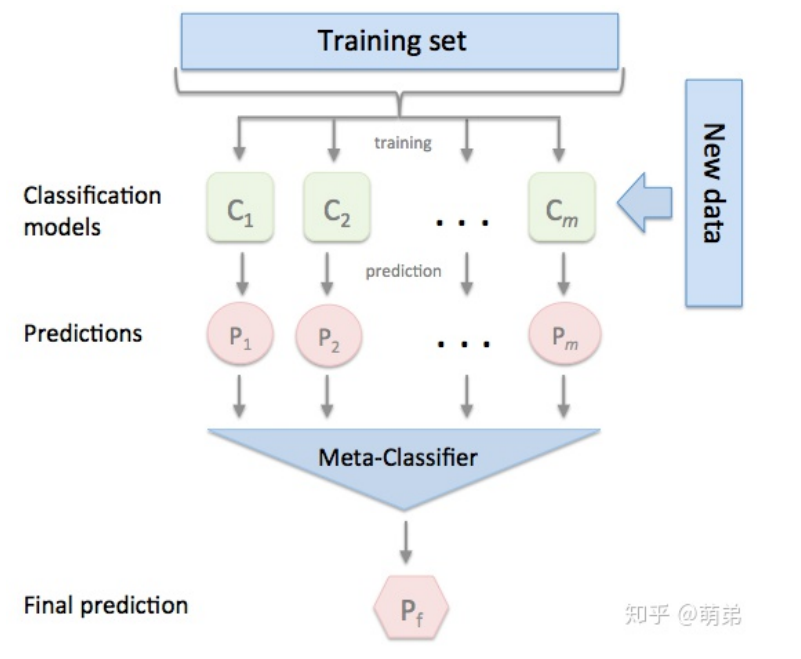## 集成学习（下）Blending集成学习算法

2021/5/12 2:56:39 人评论

1. Blending集成学习思路 Blending集成学习方式&#xff1a; (1) 将数据划分为训练集和测试集(test_set)&#xff0c;其中训练集需要再次划分为训练集(train_set)和验证集 (val_set)&#xff1b; (2) 创建第一层的多个模型&#xff0c;这些模型可以使同质的也可以是异质的&…

# 1. Blending集成学习思路

Blending集成学习方式：
(1) 将数据划分为训练集和测试集(test_set)，其中训练集需要再次划分为训练集(train_set)和验证集
(val_set)；
(2) 创建第一层的多个模型，这些模型可以使同质的也可以是异质的；
(3) 使用train_set训练步骤2中的多个模型，然后用训练好的模型预测val_set和test_set得到val_predict,
test_predict1；
(4) 创建第二层的模型,使用val_predict作为训练集训练第二层的模型；
(5) 使用第二层训练好的模型对第二层测试集test_predict1进行预测，该结果为整个测试集的结果。

bending是一种模型融合方法，对于一般的blending，主要思路是把原始的训练集先分成两部分，比如70%的数据作为新的训练集，剩下30%的数据作为测试集。第一层我们在这70%的数据上训练多个模型，然后去预测那30%数据的label。在第二层里，我们就直接用这30%数据在第一层预测的结果做为新特征继续训练即可。2400个样本的结果 ，输入测试集2000个得到K组2000个样本的结果 。Blending集成方式的优劣：

# 实例演示

``````# 加载相关工具包
import numpy as np
import pandas as pd
import matplotlib.pyplot as plt
plt.style.use("ggplot")
%matplotlib inline
import seaborn as sns
``````
``````# 创建数据
from sklearn import datasets
from sklearn.datasets import make_blobs
from sklearn.model_selection import train_test_split
data, target = make_blobs(n_samples=10000, centers=2, random_state=1, cluster_std=1.0 )
## 创建训练集和测试集
X_train1,X_test,y_train1,y_test = train_test_split(data, target, test_size=0.2,
random_state=1)
## 创建训练集和验证集
X_train,X_val,y_train,y_val = train_test_split(X_train1, y_train1, test_size=0.3,
random_state=1)
print("The shape of training X:",X_train.shape)
print("The shape of training y:",y_train.shape)
print("The shape of test X:",X_test.shape)
print("The shape of test y:",y_test.shape)
print("The shape of validation X:",X_val.shape)
print("The shape of validation y:",y_val.shape)
``````

The shape of training X: (5600, 2)
The shape of training y: (5600,)
The shape of test X: (2000, 2)
The shape of test y: (2000,)
The shape of validation X: (2400, 2)
The shape of validation y: (2400,)

``````# 设置第一层分类器
from sklearn.svm import SVC
from sklearn.ensemble import RandomForestClassifier
from sklearn.neighbors import KNeighborsClassifier
clfs = [SVC(probability = True),RandomForestClassifier(n_estimators=5, n_jobs=-1,
criterion='gini'),KNeighborsClassifier()]
# 设置第二层分类器
from sklearn.linear_model import LinearRegression
lr = LinearRegression()
``````
``````# 输出第一层的验证集结果与测试集结果
val_features = np.zeros((X_val.shape,len(clfs))) # 初始化验证集结果
test_features = np.zeros((X_test.shape,len(clfs))) # 初始化测试集结果
for i,clf in enumerate(clfs):
clf.fit(X_train,y_train)
val_feature = clf.predict_proba(X_val)[:, 1]
test_feature = clf.predict_proba(X_test)[:,1]
val_features[:,i] = val_feature
test_features[:,i] = test_feature
``````
``````# 将第一层的验证集的结果输入第二层训练第二层分类器
lr.fit(val_features,y_val)
# 输出预测的结果
from sklearn.model_selection import cross_val_score
cross_val_score(lr,test_features,y_test,cv=5)
``````

array([1., 1., 1., 1., 1.])

暂无相关的数据...